## Table of ContentOpen Access

ARTICLE

# Data Mining Based Integrated Electric-Gas Energy System Multi-Objective Optimization

Zhukui Tan1,*, Yongjie Ren1, Hua Li1, Weili Ren2, Xichao Zhou2, Ming Zeng1

1 North China Electric Power University, Beijing, 102206, China
2 State Grid Integrated Energy Service Group, Beijing, 100052, China

* Corresponding Author: Zhukui Tan. Email:Energy Engineering 2022, 119(6), 2607-2619. https://doi.org/10.32604/ee.2022.019550

## Abstract

With the proposal of carbon neutrality, how to improve the proportion of clean energy in energy consumption and reduce carbon dioxide emissions has become the important challenge for the traditional energy industry. Based on the idea of multi-energy complementarity, a typical integrated energy system consisting of electric system and gas system is constructed based on the application of power to gas (P2G) technology and gas turbine in this paper. Furthermore, a multi-objective optimization model with economic improvement, carbon emission reduction and peak-load shifting as objectives is proposed, and solved by BSO algorithm. Finally, a typical power-gas coupling system is selected as an example to verify the effectiveness of the model. The results showed that the proposed multi-objective optimization model based on BSO algorithm can better play the complementary characteristics of the electric and gas system, and significantly improve the comprehensive benefits of system operation.

## Keywords

1  Introduction

At present, China is undergoing profound energy reform . China vigorously advocates the concept of green development. Energy consumption supply, energy structure transformation and energy system form all present the development trend of network, intelligence and digitalization . After the double carbon target was proposed, the development of clean energy accelerated, and the connection between different energy systems became closer. At the same time, the development of integrated energy services improves energy efficiency, reduces energy consumption costs, and promotes competition and cooperation .

The core problem of integrated energy system development lies in system operation optimization , which has been studied by experts and scholars at home and abroad. Li et al.  established a model for optimal scheduling of thermal and electric energy integrated energy system, and verified the benefits of the model in fuel saving, clean energy consumption, capacity saving and other aspects with actual grid data based on considering the reserve benefits of heat storage tank and operation strategy. Cong et al.  constructed the asynchronous operation mechanism and framework of the power-natural gas joint market with the participation of comprehensive energy service providers, established a two-stage nested two-layer optimization model of the integrated energy system, which can improve the operation efficiency, reliability and flexibility of the integrated energy system. Natural gas has the advantages of high energy efficiency, low emissions and strong controllability , so it plays a crucial role in the development of integrated energy system. However, the uncertainty of natural gas pressure energy and the random fluctuation of pipe network load limit the improvement of pressure energy utilization efficiency in the integrated energy system . There are some limitations in the current research, the coupling depth of electricity and gas is not enough. Natural gas is only taken as a primary energy, the deep coupling between electricity and gas and the constraint of natural gas network are not considered.

In view of this situation, basis on the electric power network and natural gas network operation characteristic, this paper puts forward a kind of electricity-gas coupling integrated energy system multi-objective optimization scheduling model which considering economic benefit, environmental benefit and peak-load shifting benefit, and provides theoretical support for the comprehensive benefit improvement of integrated energy system.

2  Power-Gas Coupling System IES Multi-Objective Optimization System

2.1 Power-Gas Coupling System

As shown in Fig. 1, the power-gas coupling system consists of an electric power network and a natural gas network, and realizes the conversion of electric energy through P2G and gas turbine. P2G can convert surplus wind power output into natural gas. Therefore, the electric energy input of P2G can be regarded as the charge of the power network, while its natural gas output can be regarded as the source of the natural gas network. On the contrary, the gas turbine converts natural gas into electricity, so the natural gas input of the gas turbine is regarded as the charge of the natural gas network, and its electrical energy output is regarded as the source of the power network . The multi-objective optimal scheduling of power-gas coupling system is to achieve the optimal operation state of the entire power-gas coupling system by coordinating the economic, low-carbon and peak-load shifting objectives on the premise of satisfying the load supply [10,11] and the security constraints of power and natural gas networks.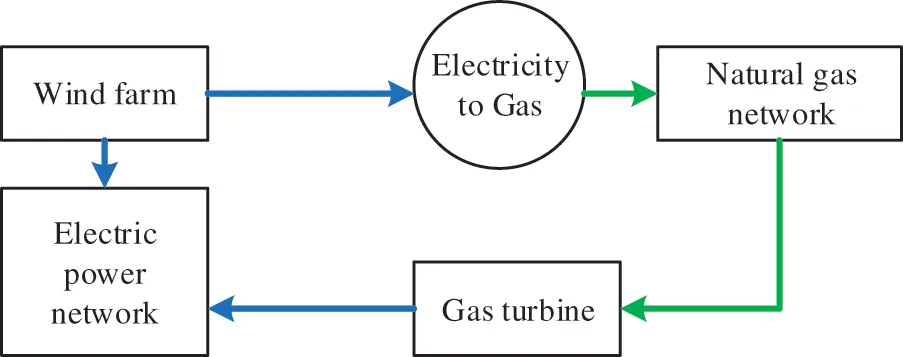Figure 1: Frame diagram of power-gas coupling system

2.2 Optimization Objectives

(1) Economic objective

The economic objective of the multi-objective optimal scheduling of the electrical coupling system is to minimize the operating cost of the system , including the cost of coal-fired power generation, the cost of natural gas purchase and the cost of wind curtailment.

f1=tT[iΩGC(aiPGC,it2+biPGC,it+ci)+iΩNgiFN,it+iΩWδi(PW0,itPW,it)](1)

where: t is the scheduling period; T is the total scheduling period; ΩGC, ΩNΩW are coal-fired units, gas sources and wind farms. PGC,it is the active output of coal-fired unit i in time period t; ai, bi, ci is the power generation cost coefficient of coal-fired unit i; gi is the natural gas purchase cost coefficient of gas source i; FN,it is the output gas flow of gas source i in period t; PW0,it and PW,it are the available active power and actual active power output of wind farm i in period t. δi is the wind curtailment penalty coefficient of wind farm i.

(2) Carbon emission objective

The carbon emission of the power-gas coupling system in the whole operation cycle is the difference between the sum of CO2 emitted by coal-fired units and gas turbines and CO2 consumed by power-gas conversion.

f2=tT[IΩGαiPG,it2+βiPG,it+γi]iΩP2GμP2GFP2G,it(2)

where: ΩG is the set of units; PG,it is the active power output of unit i in period t; αi, βi, γi are carbon emission coefficients of unit i. ΩP2G is the set of P2G; FP2G,it is the output gas flow of P2G equipment i; μP2G is the CO2 consumed per unit gas output, which is 1.8 kg/m3 in this paper.

The goal of multi-objective optimal scheduling of power-gas coupling system is to minimize net power load variance.

{f3=1Tt=1T(PnettPnet,av)Pnett=iΩENPD,it+iΩP2GPP2G,itiΩGTPGT,itiΩWPW,it(3)

where: Pnett is the net power load in period t; Pnet,av is the average value of net load within the scheduling period; ΩEN is the power network node set; PD,it is the actual active load of node i; PP2G,it is the active power input of P2G equipment i; ΩGT is the gas turbine set; PGT,it is the active output of gas turbine i.

2.3 Constraints

The constraints of multi-objective optimal scheduling of power-gas coupling system include power subsystem constraints, natural gas subsystem constraints and coupling constraints of their networks [13,14].

2.3.1 Power Network Constraints

Power network constraints include node power balance constraints, unit active power output constraints, unit climbing constraints, node voltage constraints and branch capacity constraints, as follows:

{PG,itPD,it=0PG,iminPG,itPG,imax,iΩGrd,iPG,itPG,it1ru,i,iΩGViminVitVimax,iΩENSlminSltSlmax,lΩEL(4)

where: the variables labeled max and min are the upper and lower limits of the corresponding variables, respectively; ΩEN and ΩEL are nodes and branch sets of power network, respectively. PG,it is the active power injection of generator i; PD,it is the active load of node i; rd,i and ru,i are the downward and upward climbing rates of unit i, respectively; Vit is voltage of node i; Slt is the apparent power of branch l.

2.3.2 Natural Gas Network Constraints

Like the power network, the natural gas network can also be regarded as composed of source, network, load, storage, etc. . The natural gas provided by the gas source is transmitted to the user through the pressure station and natural gas pipeline. At the same time, the gas storage tank can also play the role of storing natural gas and replacing the gas source .

(1) Gas source

The gas source is similar to a generator, injecting natural gas into the natural gas network, and each gas source must meet the output flow limit.

FN,iminFN,itFN,imax(5)

where: FN,imax and FN,imin are the upper and lower limits of the output flow of gas source i, respectively.

(2) Pipelines

The transmission flow in natural gas pipeline is mainly related to the pressure of the nodes on both sides of the pipeline and the transmission characteristics of the pipeline .

Fijt={hijpi2pj2,pipjhijpi2pj2,pi<pj(6)

where: Fijt is the pipeline transmission flow of i and j on both sides; hij is the transmission characteristic constant of pipeline ij; pi and pj are the pressure of nodes i and j, respectively. In addition, each node of the natural gas network must meet the upper and lower pressure limits.

piminpitpimax(7)

where: pimax and pimin are the upper and lower limits of pressure of nodes, respectively.

(3) Pressure station

Due to friction resistance in natural gas pipelines, part of the energy will be lost in the transmission process, resulting in pressure drop. Therefore, a certain number of pressure stations should be installed in the natural gas network to ensure reliable transmission of natural gas.

Pressure stations mainly use compressors to increase the pressure. The compressors consume natural gas, and their consumption is related to the natural gas flow through the compressors and the compression ratio:

τcom,kt=hcom,kFknt(Rkt1)(8)

RkminRkt=pktpmtRkmax(9)

where: τcom,kt is the gas flow consumed by compressor k in period t; hcom,k is the consumption coefficient of compressor k; Fknt is the gas flow from the outlet of compressor k to the downstream node n in period t; Rkt is the pressure ratio of compressor k at time period t; pkt and pmt are compressor outlet pressure and compressor upstream node m pressure, respectively. Rkmax and Rkmin are the upper and lower pressure ratios of compressor k.

(4) Storage tank

When the natural gas load fluctuates greatly or the natural gas network breaks down, the gas storage tank can be used as an alternative gas source to ensure the reliable supply of natural gas . Similar to power network energy storage devices, gas storage tanks should meet the following constraints:

{QS,it=QS,it1+FS,iin,tFS,iout,tQS,iminQS,itQS,imax0FS,iin,tFS,iin,max0FS,iout,tFS,iout,max(10)

where: QS,it is the gas storage capacity of gas storage tank i in time period t; FS,iin,t and FS,iout,t are the input and output gas flows of gas storage tank i, respectively. QS,imax and QS,imin are the upper and lower limits of gas storage capacity of gas storage tank i. FS,iin,max and FS,iout,max are the upper limits of input and output gas flow of gas storage tank i, respectively.

(5) Flow balance

Similar to node power balance constraint in power network, natural gas network also needs to meet node flow balance constraint:

wt+AS(FSout,tFSin,t)+AP2GFP2GtAFftTτt=0(11)

where: wt is the net gas flow of nodes (gas source node is positive, load node is negative); AS, AP2G and AF are node-gas storage tank correlation matrix, node-electricity to gas correlation matrix and node-pipeline correlation matrix of natural gas network, respectively. FSout,t and FSin,t are the output and input gas flow matrices of the gas storage tank, respectively. FP2Gt is the electric-gas output gas flow matrix; ft is the pipeline flow vector; T is node-pressure station correlation matrix; τt is the pressure station consumption vector.

2.3.3 Coupling Constraints

Coupling constraints between power network and natural gas network include P2G constraint and gas turbine constraint.

(1) P2G

P2G constraint including power transformation equation and maximum output gas flow restrictions.

{FP2G,it=ηP2G,iPP2G,it/CgFP2G,itFP2G,imax(12)

where: ηP2G,i is the conversion efficiency of P2G equipment i; Cg is the calorific value of natural gas, generally 39 MJ/m3; FP2G,imax is the upper limit of output gas flow for the P2G equipment i.

(2) Gas turbine

Gas turbine constraint also include power conversion equations and output active power limits [19,20]. Since the output active power limit is included in the unit active power output constraint of Eq. (13), only the power conversion equation is described here.

FGT,it=h2,i(PGT,it)2+h1,iPGT,it+h0,i(13)

where: FGT,it is the input gas flow of gas turbine i in time period t; PGT,it is the active output of gas turbine i in time period t; h2,i, h1,i and h0,i are the consumption coefficients of gas turbine i.

3  Solving of the Optimization Problem

BSO (Brain Storm Optimization) is a swarm intelligence optimization algorithm, compared with other algorithms, such as genetic algorithm, PSO algorithm, etc., BSO algorithm can use one or two “old” individuals or groups to generate new individuals, new groups, and thus out of the original search range, and effectively avoid falling into the local optimum. The core idea is to simulate the crowd to propose a large number of solutions to problems. Each solution is regarded as a feasible solution, and the process of obtaining the optimal solution through discussion and integration. It has the advantages of good stability, high accuracy, and fast convergence . In view of this, this paper uses the BSO algorithm to solve the multi-objective optimization problem of electrically coupled system operation. The solution process is shown in Fig. 2. The main steps of the solution are as follows :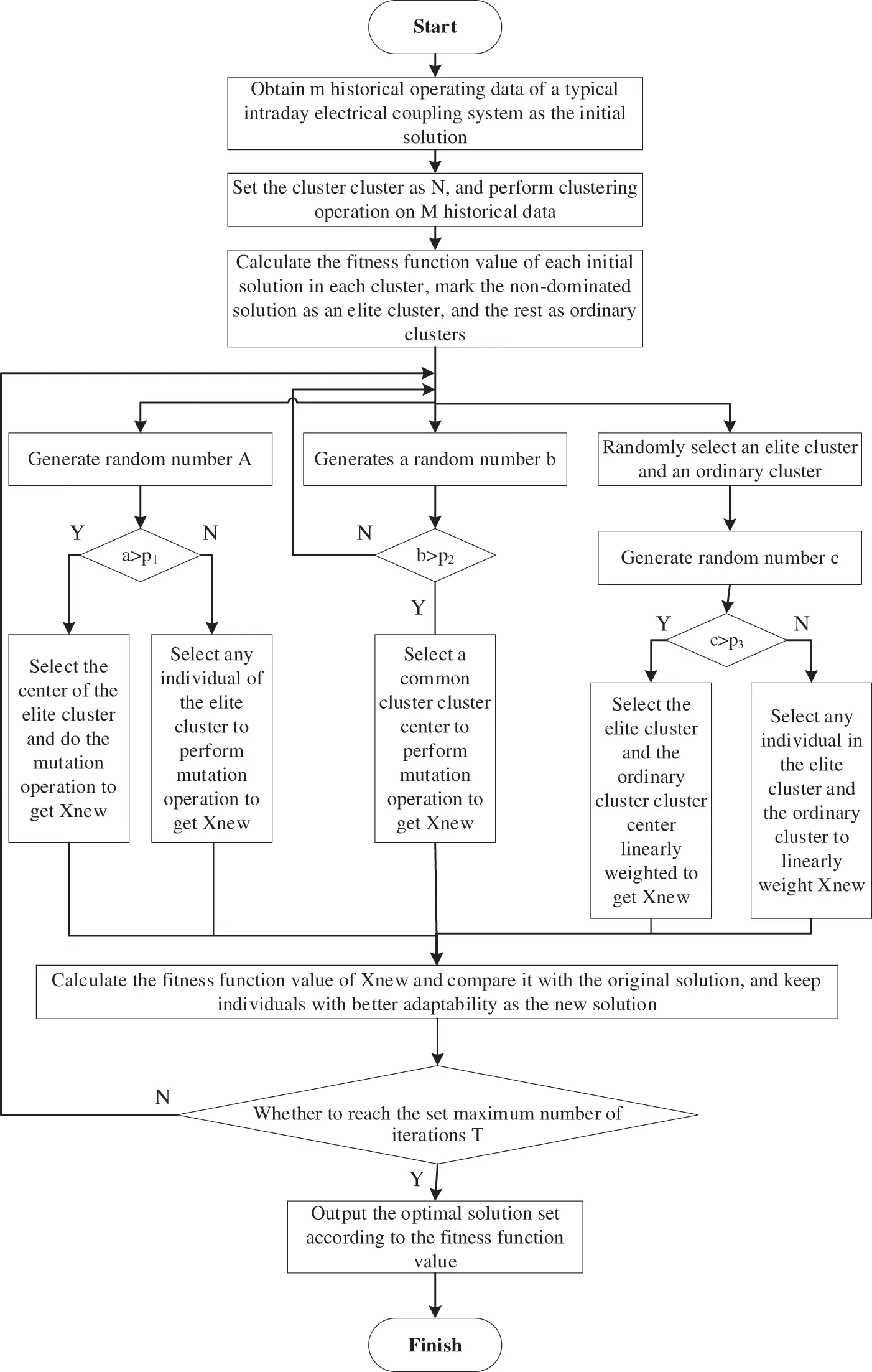Figure 2: Schematic diagram of multi-objective optimization solution flow of electrical coupling system based on BSO

(1) Initial solution population is generated

Obtain m pieces of historical data of a typical intraday electrical coupling system as the initial feasible solution of the optimization problem. Perform clustering operations on m initial feasible solutions to obtain n clusters of initial solution populations, and calculate the fitness function value of each initial feasible solution. The clusters containing at least one non-dominated solution are marked as elite clusters, and the clusters without non-dominated solutions are marked as ordinary clusters.

(2) Generation of new solutions

In BSO algorithm, new solutions are generated in the following ways:

(1) Select a random elite cluster and generate a random number a(0,1), if a is greater than the set probability threshold p1, the cluster center of elite cluster is selected for mutation operation to obtain a new individual. On the contrary, an individual is randomly selected from the elite cluster for mutation operation to obtain a new individual.

(2) Generate a random number b(0,1), if b is greater than the set probability threshold p2, the cluster center of a common cluster is randomly selected to obtain a new individual through mutation operation.

(3) Select an elite cluster and a common cluster, and generate random number c(0,1), if c is greater than the set probability threshold p3, the cluster centers of elite clusters and ordinary clusters are selected to obtain new individuals through linear weighting. On the contrary, the ordinary individuals in the elite cluster and the ordinary cluster are selected and the new individuals are obtained by linear weighting.

(4) Calculate the fitness function value of the new individual and compare it with the original solution, and keep the individual with good fitness function value as the new solution.

Among them, the mutation operation is achieved by adding random values to selected individuals, and the mutation operation is as follows:

Xnew=Xselected+αN(μ,σ). In the above formula, Xnew is the new individual from the mutation operation; Xselected is the selected individual of the original solution; α is the coefficient of the Gaussian function, used to control the amplitude of the disturbance; N(μ,σ) is a Gaussian distribution with mean μ and variance σ.

(3) Solution generation of multi-objective optimization problem

Repeat (1) and (2) until the number of iterations reaches the set value T, and then output the optimal solution set according to the fitness function value.

4  Empirical Example and Analysis

4.1 Case Design

This article takes the modified IEEE39-node electric power network and 20-node natural gas network interconnection electrical coupling system as the object, takes one day as the analysis period and 1 h as the scheduling period, to analyze and test the effectiveness of the model proposed in this article. The schematic diagram of the electrical coupling system is shown in Fig. 3.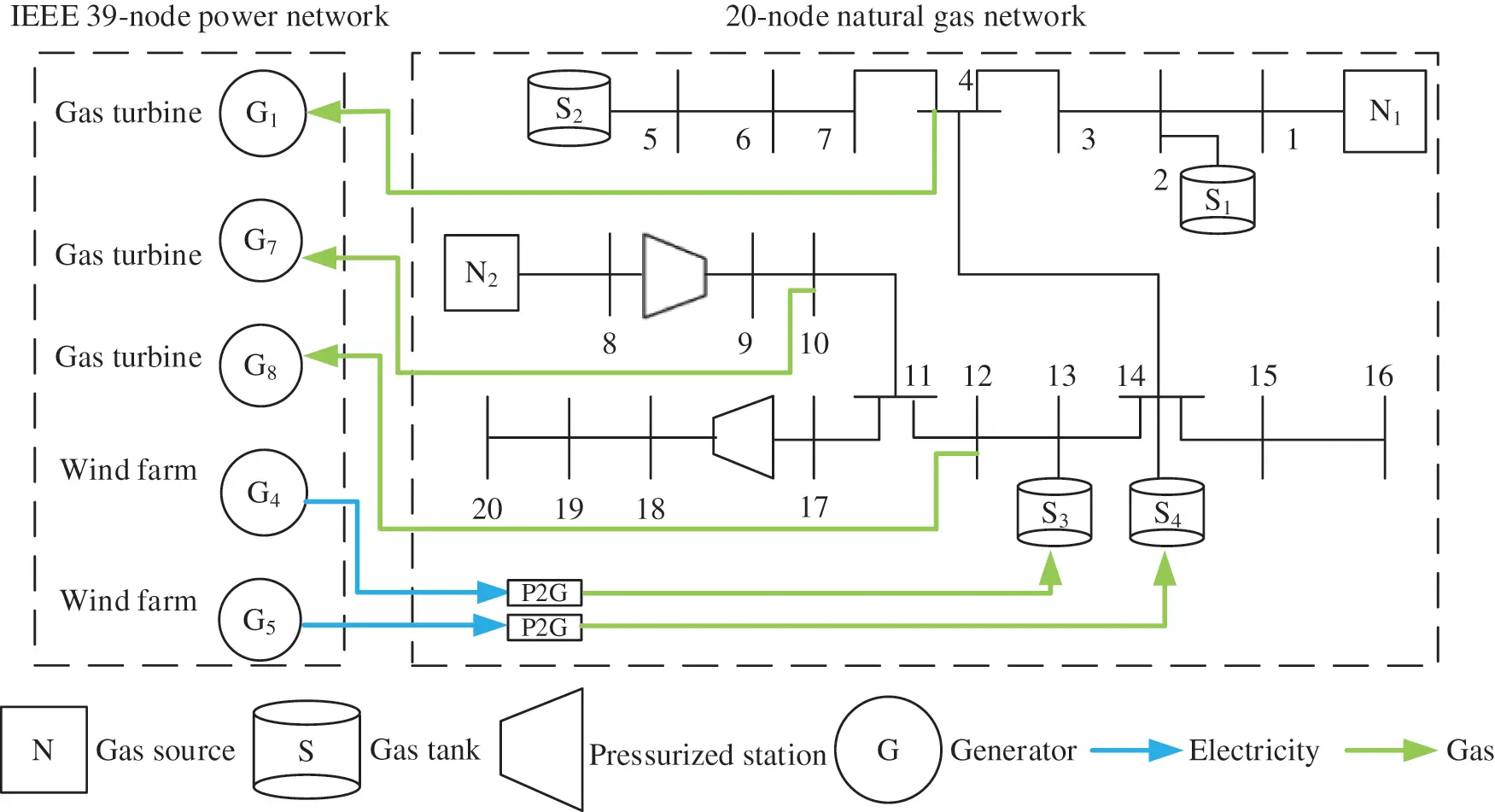Figure 3: The schematic diagram of the electrical coupling system

The load of each node of the power sub-network becomes 80% of the IEEE 39-node power network standard test network, units G1, G7 and G8 are gas turbines, connected to load nodes 4, 10 and 12 of the natural gas network, units G4 and G5 are wind farms with a rated power of 600 MW, both wind farms are equipped with P2G equipment and are connected to natural gas network gas storage tanks S3 and S4. The wind farm abandonment penalty coefficient is both US\$ 50(MW·h). The remaining units in the power network are coal-fired units. The parameters of gas turbine and coal-fired units are shown in Tables 1 and 2. The natural gas sub-network contains 20 nodes and 19 pipelines, parameters of gas source and storage are shown in Tables 3 and 4, respectively.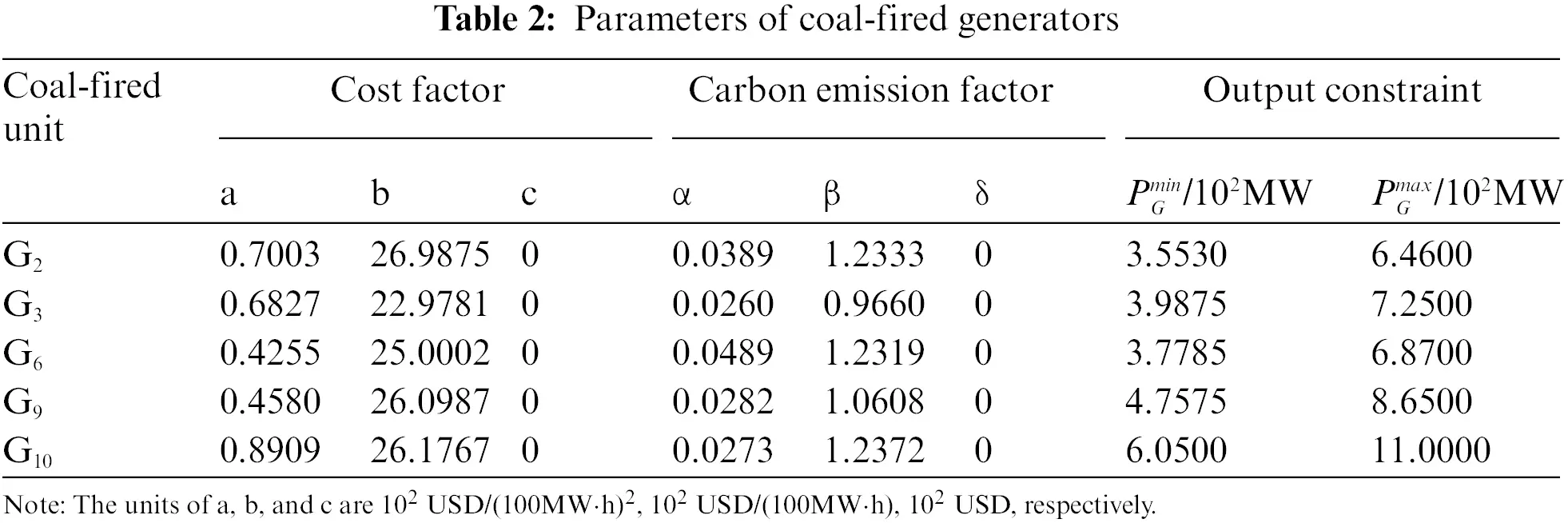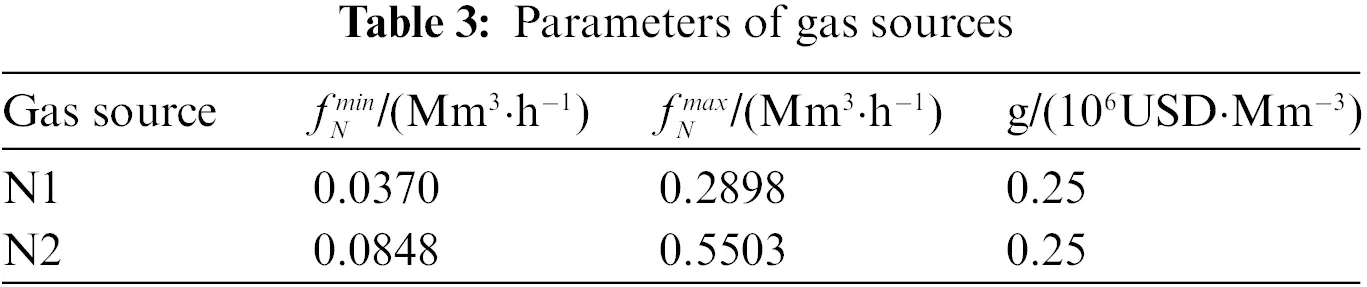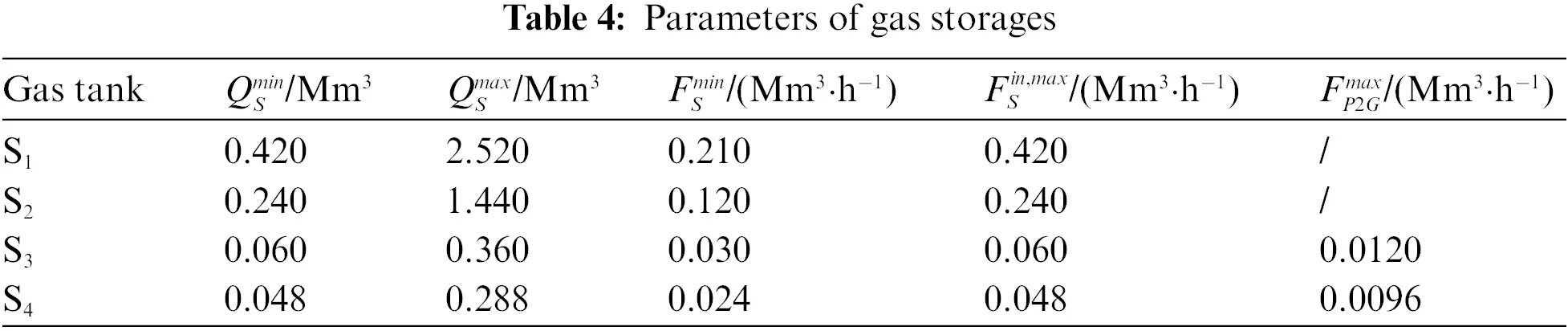In the dispatch day, the available wind farm power, electrical load and gas load of the system are shown in Fig. 4.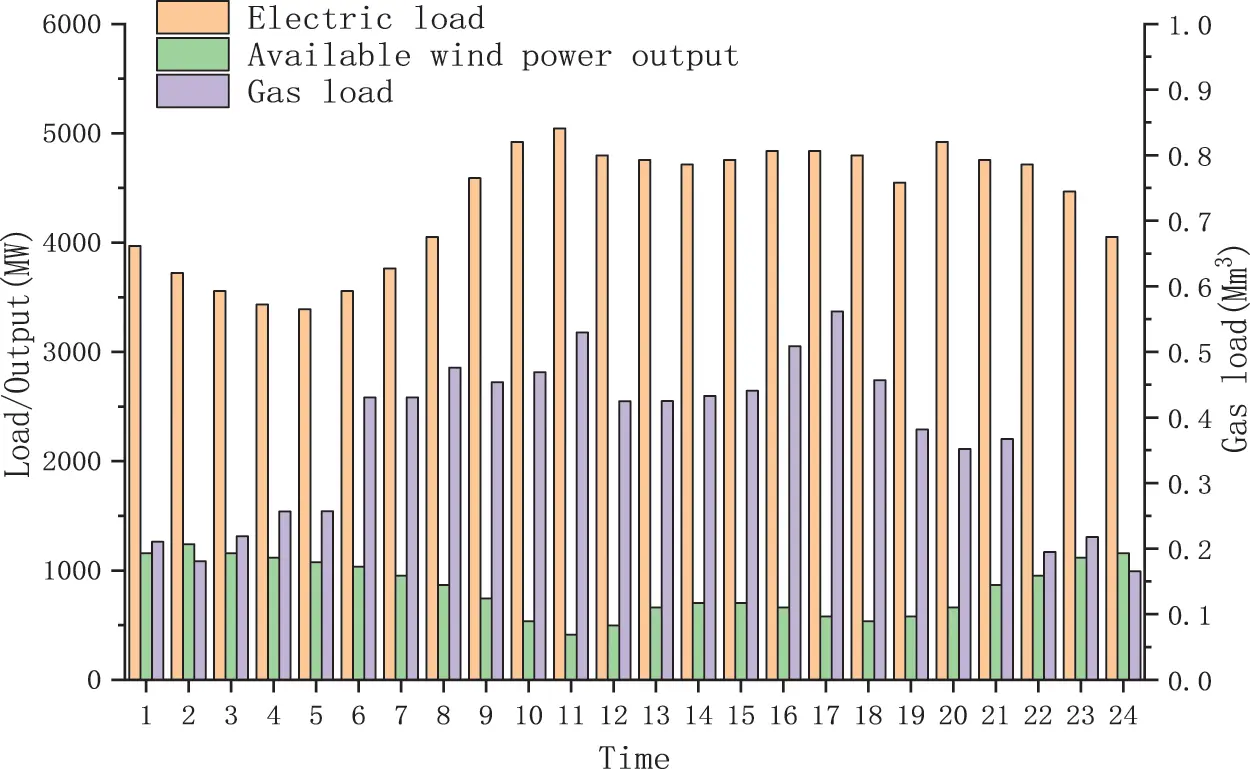Figure 4: Available wind power, electric and gas load

In order to fully illustrate the superiority of the multi-objective optimal scheduling of the electric-to-gas-electric coupling system in this article, two scenarios are designed for the calculation example: one is a single-objective optimization scenario that targets economy, and the other is a three-objective optimization scenario that targets economy, carbon emissions, and the comprehensive benefits of peak shaving and valley filling. In order to make full use of wind power to reduce operating costs and carbon emissions, the output of wind farms under Scenario 2 of this article is consistent with Scenario 1.

4.2 Scheduling Analysis

Make the weights of each target in Scenario 2 equal, the wind farm output corresponding to the optimal solution of the single target in Scenario 1 and the compromise solution of multiple targets in Scenario 2, gas turbine output. The injection power of electric-to-gas and the net electric load are shown in Figs. 5 and 6. The corresponding wind power consumption ratio and each target value are shown in Fig. 7.Figure 5: Simulation results in scene 1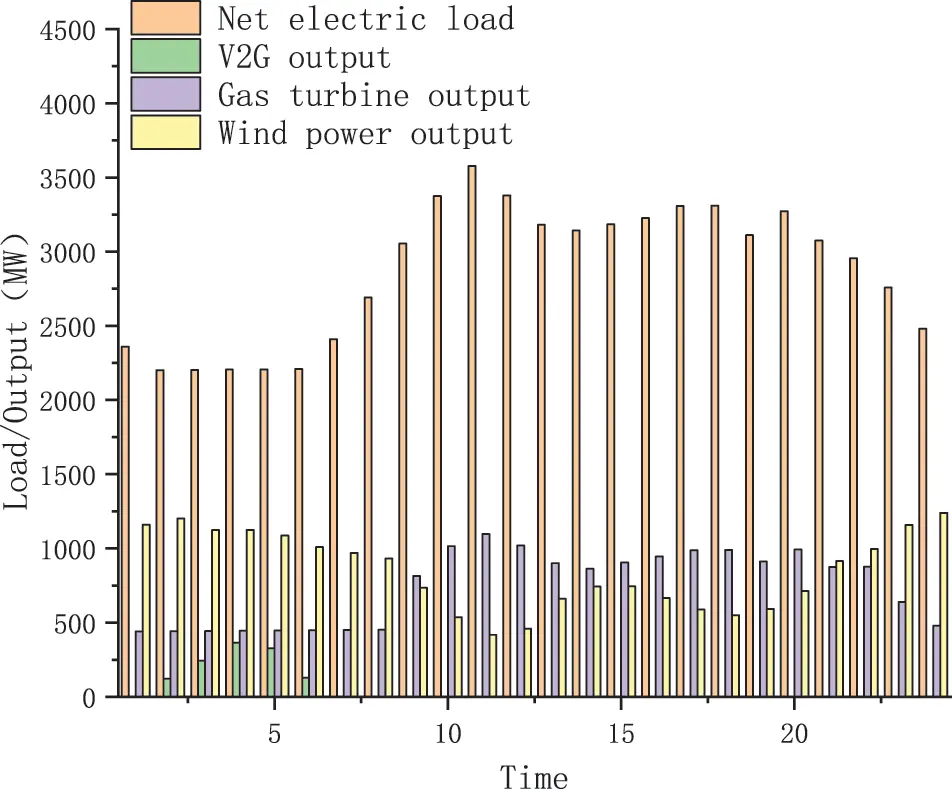Figure 6: Simulation results in scene 2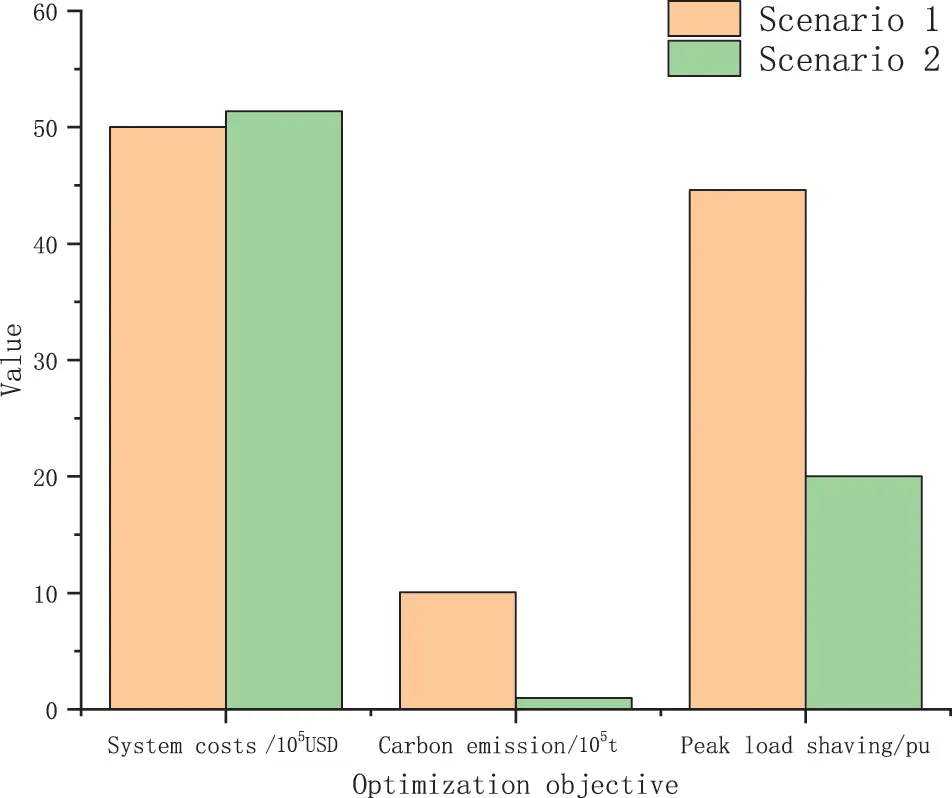Figure 7: Dispatch rates of wind power and objective values

It can be seen from Fig. 5 that in Scenario 1, the wind farm power is more surplus from 01:00–07:00, and the power load is at a low stage during this period. Therefore, P2G equipment works from 01:00–07:00, which can convert surplus wind energy into natural gas for storage, thereby improving the system’s ability to absorb wind energy. In addition, since the price of natural gas is more expensive than that of coal, gas turbines operate at almost the minimum output and only increase their output at 11:00 during the peak power consumption period to make up for the shortage of power supply for coal-fired units. In Scenario 2, due to the comprehensive consideration of economy, carbon emissions, and peak-shaving and valley-filling, the three target P2G equipment and gas turbines work together to reduce system carbon emissions and smooth the net power load curve, subject to P2G efficiency, additional P2G conversion will increase system operating costs and carbon emissions. Therefore, the injection power of the P2G equipment in Scenario 2 does not change significantly compared with Scenario 1, while the output of gas turbine has a significant change compared with Scenario 1. The increase in gas turbine output can not only significantly reduce the carbon emissions of the system, but also play a role in peak shaving, thereby smoothing the net power load curve of the system. It can be seen from the data in Fig. 7 that in Scenario 2, although the economic target is increased by 2.72% compared to Scenario 1, the remaining carbon emission targets and peak-shaving and valley-filling targets are improved by 8.10% and 55.09%, respectively. The superiority of multi-objective optimal dispatching of the electrical coupling system that takes into account economy, carbon emissions, and peak-to-valley-filling targets.

5  Conclusion

Based on considering the operation characteristics and constraints of power system and natural gas system, this paper proposes a multi-objective optimization model of electric coupling integrated energy system, which considers economic benefits, environmental benefits, and peak-cutting and valley filling utility, based on electricity to gas technology and gas turbine technology and uses BSO algorithm to solve it. In this paper, the effectiveness of the model is further verified by an example, the results show that compared with the traditional scheduling optimization based on economy, the proposed model can give better play to the comprehensive benefits of the electric-coupling integrated energy system.

Funding Statement: This work was supported by the State Grid Corporation Technology Project (Research and Application of Integrated Energy System Regulation Technology of Power Source, Grid, Load and Storage Interaction (No. SGFJJY00GHJS1900066)).

Conflicts of Interest: The authors declare that they have no conflicts of interest to report regarding the present study.

## References

1. Zhou, X. X., Zeng, R., Gao, F., & Qu, L. (2017). Development status and prospects of the energy internet. Scientia Sinica (Informationis), 47(2), 149-170. [Google Scholar]
2. Li, K., & Zhang, Y. K. (2019). Development situation and opportunities of China’s energy saving market. China’s Energy, 41(12), 28-32. [Google Scholar]
3. Meng, H. (2019). Development status and countermeasures on energy disruptive technologies. Global Science, Technology and Economy Outlook, 34, 71-78. [Google Scholar]
4. Yuan, C. G. (2021). Research on optimal operation and market bidding strategy of electric-gas integrated energy system (Master Thesis). Northeast Electric Power University, China.
5. Li, J., Li, X. F., Zhang, N., Zhang, Y., Lv, Q. (2021). An optimal dispatch model of the electricity-heat integrated energy system considering the reserve benefits of the heat storage. Power System Technology, 45(10), 3851–3859.
6. Cong, H., Wang, X., & Jiang, C. W. (2019). Strategies of optimal operation of integrated energy system in asynchronous market environment. Power System Technology, 43(9), 3110-3120. [Google Scholar]
7. Qiu, B., Zhang, Z. C., Wang, K., Mu, H. B., & Yang, Z. (2022). Pressure energy generation of natural gas pipeline considered optimal dispatching research for IES. Power System Technology, 46(4), 1457-1464. [Google Scholar] [CrossRef]
8. Cao, B., Lv, G. Y., Wang, N., & Jia, D. X. (2021). Research and application of demand response based on optimal scheduling of integrated energy system. Power Demand Side Management, 23(4), 45-50. [Google Scholar]
9. Zhang, T. (2021). Study on coordination and optimization of supply and demand benefits of integrated energy system (Master Thesis). Zhejiang University, China.
10. Zhang, J., Fan, H., Zhang, X. J., Dong, Y., & Li, M. Y. (2021). Optimal model of cross-regional integrated energy system dispatching considering risk cost. Thermal Power Generation, 2021(8), 121-130. [Google Scholar] [CrossRef]
11. Xu, J., Xu, K., Wang, S. J., Li, J., Zhang, X. F. (2021). A multi-energy flow calculation method based on discrete iteration of electricity-gas integrated energy systems. Proceedings of the CSU-EPSA, 34(1), 114–120. DOI 10.19635/j.cnki.csu-epsa.000783. [CrossRef]
12. Wang, S. (2021). Reliability evaluation of integrated energy systems considering flexibility on demand side (Ph.D. Thesis). Zhejiang University, China.
13. Shuai, X. Y., Wang, X. L., & Huang, J. (2021). Optimal configuration of shared energy storage capacity under multiple regional integrated energy systems interconnection. Journal of Global Energy Interconnection, 4(4), 382-392. [Google Scholar]
14. Fang, C., Zhang, Y., Liao, W., Shi, S. S., & Liu, D. (2021). Benefit evaluation of energy storage in multi-energy collaborative optimization of regional energy internet. Electric Power Construction, 42(5), 48-56. [Google Scholar]
15. Jiang, Y., Li, X. F., Gao, D. C., Duan, R. Q., Zhou, H. et al. (2021). Combined forecasting of electricity and gas load for regional integrated energy system. Electrical Measurement & Instrumentation. https://kns-cnki-net.webvpn.ncepu.edu.cn/kcms/detail/23.1202.TH.20210429.1059.008.html.
16. Zhou, S. C., Guo, Z. X., Cheng, X., Chen, L., & Zuo, Z. M. (2021). Planning approach of P2G stations for integrated energy system of electricity-gas interconnection. Guangdong Electric Power, 34(2), 36-44. [Google Scholar]
17. Wang, Y. B., Quan, Z. H., Jing, H. R., Wang, L. C., & Zhao, Y. H. (2021). Thermodynamic analysis and operation optimization of multi energy complementary energy storage system. CIESC Journal, 72(5), 2474-2483+2906. [Google Scholar]
18. Qu, J. T. (2020). Analysis of integrated energy system planning based on multi-energy complementation. Electric Engineering, 2020(24), 43-46. [Google Scholar]
19. Chen, Y. (2020). Multi-energy complementary clean energy collaborative optimization scheduling analysis. China New Telecommunications, 22(14), 134-135. [Google Scholar]
20. Yang, Y. P., Duan, L. Q., Du, X. Z., Wang, X. D., & Xu, C. (2020). Research foundation and prospect on distributed energy system with the complementation of multiple energy sources. Bulletin of National Natural Science Foundation of China, 34(3), 281-288. [Google Scholar]
21. Wu, Y. L., Fu, Y. L., Li, G. T., & Zhang, Y. C. (2020). Many-objective brain storm optimization algorithm. Control Theory & Applications, 37(1), 193-204. [Google Scholar]
22. Guo, Y. N., Jiang, D. Z., Wang, R. R., Gong, D. W. (2021). Structural design of heat exchanger plate with wide-channel based on multi-objective brain storm optimization. Control and Decision. https://kns.cnki.net/kcms/detail/detail.aspx? FileName=KZYC2021070100W&DbName=CAPJ2021.

Tan, Z., Ren, Y., Li, H., Ren, W., Zhou, X. et al. (2022). Data Mining Based Integrated Electric-Gas Energy System Multi-Objective Optimization. Energy Engineering, 119(6), 2607–2619.This work is licensed under a Creative Commons Attribution 4.0 International License , which permits unrestricted use, distribution, and reproduction in any medium, provided the original work is properly cited.

View

Like

## Related articles

• Taihong Liu, Dingchen Wu, Fei...
• Fahd N. Al-Wesabi, Hadeel Alsolai,...
• Ibrahim M. Mehedi, Ubaid M. Al-Saggaf,...
• S. Neelakandan, A. Arun, Raghu...
• Yu Xue , Yan Zhao